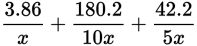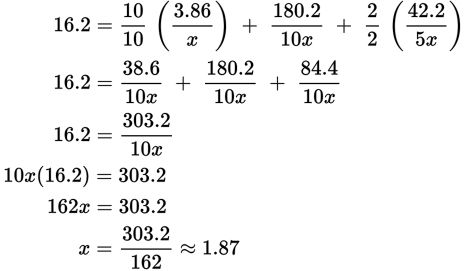# SAT Math Multiple Choice Question 510: Answer and Explanation

### Test Information

Question: 510

15.The Ironman Triathlon originated in Hawaii in 1978. The format of the Ironman has not changed since then: it consists of a 3.86-km swim, a 180.2-km bicycle ride, and a 42.2-km run, all raced in that order and without a break. Suppose an athlete bikes 10 times as fast as he swims and runs 5 times as fast as he swims. The variable x in the expression above represents the rate at which the athlete swims, and the whole expression represents the number of hours that it takes him to complete the race. If it takes him 16.2 hours to complete the race, how many kilometers did he swim in 1 hour?

• A. 0.85
• B. 1.01
• C. 1.17
• D. 1.87

Explanation:

D

Difficulty: Hard

Category: Passport to Advanced Math / Exponents

Strategic Advice: The key to answering this question is deciding what you're trying to find. The question tells you that x represents the athlete's swim rate, and you are looking for the number of kilometers he swam in one hour—these are the same thing. If you find x (in kilometers per hour), you will know how many kilometers he swam in one hour.

Getting to the Answer: Set the equation equal to the total time, 16.2, and solve for x. To do this, write the variable terms over a common denominator, 10x, and combine them into a single term. Then cross-multiply and go from there.